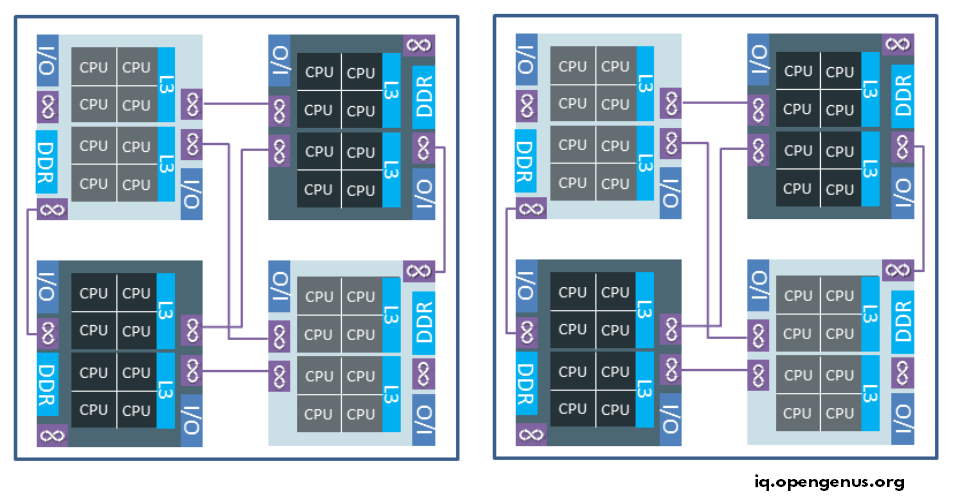×

Search anything:

# KMP_HW_SUBSET [complete guide]

#### Linux C++Get this book -> Problems on Array: For Interviews and Competitive Programming

KMP_HW_SUBSET is an OpenMP environment variable that is used to control the distribution of hardware threads across machine topology. This gives finer control compared to OMP_NUM_THREADS.

1. When to use KMP_HW_SUBSET?
2. How to use KMP_HW_SUBSET?

## When to use KMP_HW_SUBSET?

KMP_HW_SUBSET should be used in the following cases:

• When a part of the system is used and we want to ensure the load is equally distributed across the machine topology.
• We want to have finer control over the hardware threads being used.
• Get more performance compared to OMP_NUM_THREADS and default settings.

It is advised not to use OMP_NUM_THREADS when KMP_HW_SUBSET is being used. If conflicting values are placed in the two environment variables, the behaviour can be unexpected.

## How to use KMP_HW_SUBSET?

KMP_HW_SUBSET can be used to control threads across different layers of a machine topology. The different layers in a machine topology can be:

• Socket
• NUMA domain
• Cores

Following image summarizes the machine topology:The above image has:

• 2 sockets
• Each socket has 4 NUMA nodes
• Each NUMA node has 8 CPU cores
• Each CPU core may have 1 or 2 threads

Note the topology varies across system so you need to check topology of current system to use KMP_HW_SUBSET effectively.

Using KMP_HW_SUBSET, we can specify how many threads to use in total and how the threads are distributed across different layers of machine topology.

For example, consider the following command:

``````export KMP_HW_SUBSET=2s,3c,1t
``````

The above command will allow the system/ OpenMP to use:

• 2 sockets
• 3 cores in each socket
• 1 thread in each core
• In total, 6 threads (2 * 3 * 1) will be used.
• We have not specified any NUMA node so in runtime, any NUMA node can be used.
• This is assuming that the system has no NUMA node design.

The total number of threads will be same if we set the following using OMP_NUM_THREADS but the distribution will be random in OMP_NUM_THREADS and in case of KMP_HW_SUBSET, the distribution will have a pattern:

``````export OMP_NUM_THREADS=6
``````

We can specify NUMA nodes as well (if system supports it) with n option:

``````export KMP_HW_SUBSET=2s,4n,3c,1t
``````

The above command will allow the system/ OpenMP to use:

• 2 sockets
• 4 NUMA nodes in each socket
• 3 cores in each NUMA node
• 1 thread in each core
• In total, 24 threads (2 * 4 * 3 * 1) will be used.
• We have not specified any NUMA node so in runtime, any NUMA node can be used.

If an option is not specified, it is assumed by the system that all available resources in the skipped layer is used. For example, in the following command:

``````export KMP_HW_SUBSET=2s,3n,1t
``````

The core option is skipped so all cores in a NUMA node will be used. In total, 48 threads (2 * 3 * 8 * 1) will be used.

We have specify two more options seperated by colon (:):

• Core type: The design of the core. For example: intel_core or intel_opengenus
• Core efficiency: Large the efficiency, better is the performance. Value can be in the range of 0 to Number of cores-1. This is added as `eff<number>`.

These are used as follows:

``````export KMP_HW_SUBSET=4c,1t:intel_core
OR
export KMP_HW_SUBSET=4c,1t:eff1
``````

Try out different combinations and check which one gives the best performance with your system.#### Geoffrey Ziskovin

Geoffrey Ziskovin is an American Software Developer and Author with an experience of over 30 years. He started his career with Haskell and has interviewed over 700 candidates for Fortune 500 companies